Home / Electric Power / Single-Phase and Three-Phase System Explained

# Single-Phase and Three-Phase System Explained

Want create site? Find Free WordPress Themes and plugins.

This guide covers single phase and three phase systems along with the Wye (Star) and Delta connections. Three phase system advantages and synchronization process are also discussed in detail.

An alternator can be designed to generate single-phase or polyphase AC voltages. Figure 1 illustrates the basic configurations used to generate single-phase, two-phase, and three-phase AC voltages.

The stator coil or coils provide the output voltage and current, and the rotor is actually a rotating electromagnet, providing both the magnetic field and relative motion.Figure 1 Generation of single-phase and polyphase voltages.

## Single Phase System

The electric service supplied to a residence normally consists of a single-phase (1Ø) voltage supply with a center tap transformer, as shown in Figure 2.

The center tap allows two different voltages (120/240 V) from the single-phase supply. This type of voltage supply is normally adequate for most lighting and power appliances in the home. It is also known as a single-phase, three-wire supply system and split-phase system. Its main advantage is that it saves conductor material over a single-ended, single-phase system.

The transformer supplying a three-wire distribution system has a single-phase input (primary) winding. The output (secondary) winding is center-tapped and the center tap connected to a grounded neutral. The secondary transformer voltage is 120 volts on either side of the center tap, giving 240 volts between the two live conductors.Figure 2 Single-phase, three-wire supply system.

Most commercial and industrial electrical installations require three-phase distribution systems. A three-phase (3Ø) voltage supply is a combination of three, single-phase voltages. The single-phase voltage supplied to residential homes is, in fact, one of the phases taken from a three-phase distribution system. As load requirements increase, the use of single-phase power is no longer practical.

## Three-phase System

• Compared to an equivalent single-phase system, the three-phase system transmits 73 percent more power but uses only 50 percent more wire.
• The power delivered by a single-phase source is pulsating, whereas the power delivered by a three-phase system is relatively constant at all times. This means that even though the power in each phase is pulsating, the total power at any instant will be relatively constant. Therefore, the operating characteristics of three-phase machines will be superior to single-phase devices with similar ratings.
• A motor or transformer may be the same physical size, but the horsepower rating of three-phase motors and the kilovolt-ampere rating of three-phase transformers are 150 percent greater than for single-phase motors and transformers.
• A three-phase distribution system can be used to supply both three-phase and single-phase service.

A three-phase alternator contains three sets of coils positioned 120 degrees apart, and its output voltage consists of three voltage waves 120 electrical degrees apart. The order in which these voltages succeed one another is called the phase rotation or phase sequence.

With reference to the three-phase voltage waveform shown in Figure 3:

• Phase A starts to rise in a positive direction at zero electrical degrees.
• 120 electrical degrees later, phase B starts to increase in a positive direction.
• Phase C follows at another interval of 120 degrees.
• The phase sequence for this system is A, B, C, A, B, C . . .
• The phase sequence may be reversed by either reversing the direction of rotation of the alternator or interchanging the connections of any two of the three wires used for the transmission of the three-phase voltage.
• If two phases of the supply to a three-phase induction motor are interchanged, the motor will reverse its direction of rotation. A phase rotation tester can be used for measuring phase rotation where three phase supplies are used to feed motors, drives, and electrical systems.Figure 3 Phase rotation in three phase system

Alternator synchronization is the process of connecting a three-phase alternator to another alternator or to a power grid. The conditions that must be meet when paralleling a three-phase alternator to another alternator or to a power grid system are:

• The phase sequence or rotation of the machine must be the same as that of the system.
• The alternator voltage must be equal to the system voltage.
• The alternator voltage must be in phase with the system voltage.
• The alternator frequency must be equal to the system frequency.

Figure 4 illustrates the method of synchronizing an alternator to a three-phase system using the three-dark lamp method. The operation of the synchronizing process is summarized as follows:

• Three lamps, one for each phase, are connected to detect when the direction of the phase rotation of the alternator is matched with that of the three-phase source.
• If all three lamps blink on and off in unison, the phase rotation of the two are matched.
• If they do not blink in unison, the two are not matched.
• When the two are in phase and synchronized with each other and the voltages match, the three lamps will be dark or off.
• At this point, the contactor paralleling contacts can be closed. When the voltage across the lamps is zero, the voltage difference between the two systems will be zero.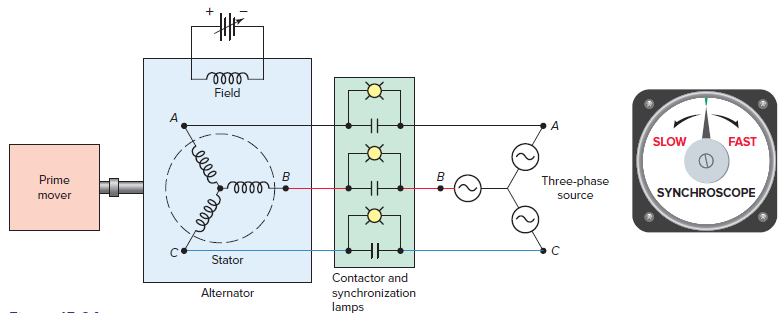Figure 4 Alternator synchronization.

In the past, synchronizing was performed manually using the three-lamp method. Today, synchronization is carried out more accurately at the exact instant of synchronism using a synchroscope. The synchroscope meter display will indicate whether there is a speed/frequency mismatch and/or a voltage mismatch between the two sources being paralleled.

Since the synchroscope meter movement can travel in a 360-degree direction, when changes are made that effect the frequency and voltage output, the effects of those changes can be observed as they are made.

Utility generators are regularly being connected and disconnected from a large power grid in response to customer demand. Such a grid is said to be an infinite bus because it contains so many generators essentially connected in parallel that neither its voltage nor its frequency can be altered. Considerable disturbance in the electrical system and damage to the alternator windings can occur if proper synchronization procedures are not followed.

The three-phase alternator contains three sets of stator coils positioned 120 degrees apart. The output from these coils is three separate voltages with the same frequency and magnitude, but 120 electrical degrees apart.

The three windings of the alternator are connected so that only three or four wires, instead of six wires, are required for the transmission of the three-phase voltage. The three sets of stator coils of the three-phase alternator may be connected in wye (also known as star) or delta, as shown in Figure 5.Figure 5 Wye and delta alternator connections in three phase system

The wye connection is made by connecting one end of each of the three-phase alternator windings together. Figure 6 shows an example of the voltages and currents in a wye-connected configuration.

The voltage across and the current through a single winding or the phase are known as the phase voltage (Ephase) and phase current (Iphase). Similarly, the voltage between and the current through the line wires are known as the line voltage (Eline) and line current (Iline).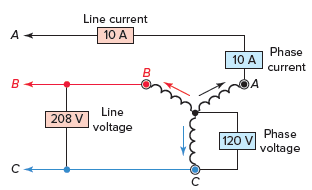Figure 6 Voltages and currents in a wye-connected configuration in three phase system

## Voltage and Current Calculation in Wye-Connection Example

Problem: Voltage and current measurements taken for a three-phase, wye-connected alternator output indicate a phase voltage of 240 volts and a line current of 30 amperes. What is the value of the line voltage and phase current?

Solution: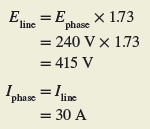Wye (Star) Connection

In a wye connection, the line current and the phase current are the same because they act in series with each other: Iline = Iphase. However, the phase voltage is less than the line voltage by a factor of the square root of 3, which equals 1.73. This is because the voltage of each phase has a 120-degree delay or shift that has to be taken into account:Or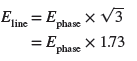The three-phase, four-wire wye system is very common and is the standard system supplied by many power utilities to commercial and industrial customers.

Figure 7 shows a four-wire, wye-connected alternator capable of supplying power to a building. It can deliver both single-phase and three-phase power and both 208 V and 120 V without the use of a transformer.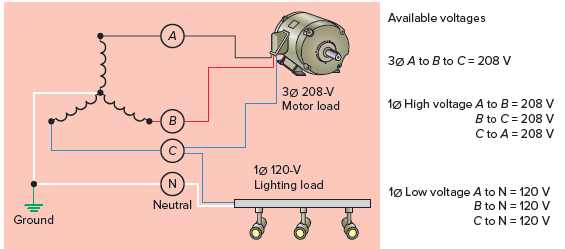Figure 7 Three-phase four-wire wye system.

The phase windings of a delta connection are all connected in series and resemble the Greek letter delta (Δ). Figure 8 shows an example of the voltages and currents in a delta-connected configuration. Since the phase windings form a closed loop, it may seem that a high current will continuously flow through the windings, even when no load is connected.

Actually, because of the phase difference between the three generated voltages, negligible or no current flows in the windings under no-load conditions.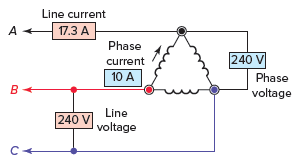Figure 8 Voltages and currents in a delta-connected configuration in three phase system

Delta Connection

In a delta connection the line voltage and the phase voltage are the same because they act in parallel with each other: Eline = Ephase. The line current and phase current, however, are different. The line current of a delta connection is higher than the phase current by a factor of 3 or 1.73. This is because the current of each phase has a 120-degree delay or shift that has to be taken into account:## Voltage and Current Calculation in Delta Connection Example

Problem: Voltage and current measurements taken for a three-phase, delta-connected alternator output indicate a line voltage of 480 volts and a line current of 100 amperes. What is the value of the voltage across each phase and the current through each phase?

Solution:Review Questions

1. What are the two single-phase voltages available from a standard residential service?
2. Compare the use of single-phase and three-phase systems with regards to the:
1. The amount of wire required for an equivalent size load.
2. The variation in the amount of power delivered to a load.
3. The physical size of three-phase and single-phase equivalent-rated motors.
3. How does phase rotation affect the direction of rotation of a three-phase induction motor?
4. What conditions must be met when connecting a three-phase alternator to a power grid system?
5. How many degrees apart are the three stator coils of a three-phase alternator positioned?
6. Name the two basic types of three-phase alternator stator coil connections.
7. How do the line-and-phase voltages and currents for a wye-connected alternator compare in value?
8. How do the line-and-phase voltages and currents for a delta-connected alternator compare in value?
9. Voltage and current measurements taken for a three-phase, wye-connected alternator indicate a line voltage of 208 volts and a line current of 20 amperes. What is the value of the phase voltage and phase current?
10. Voltage and current measurements taken for a three-phase, delta-connected alternator indicate a phase voltage of 240 volts and a line current of 10 amperes. What is the value of the line voltage and phase current?

1. 120V and 240V.
2. (a) The 3-phase system transmits 73% more power but uses only 50% more wire.
3. (b) The power delivered by a single-phase source is pulsating whereas the power delivered by a three-phase system is relatively constant.
4. (c) Three-phase motors are much smaller in size than comparable single-phase motors.
5. If two phases of the supply to a 3-phase induction motor are interchanged, the motor will reverse its direction of rotation.
6. The phase sequence or rotation of the machine must be the same as that of the system. The alternator voltage must be equal to the system voltage. The alternator voltage must be in phase with the system voltage. The alternator frequency must be equal to the system frequency.
7. 120°
8. Wye and Delta.
9. I line = I phase, E line = E phase x 1.73
10. E line = E phase, I line = I phase x 1.73
11. I phase = 20 A, E phase = 120 V
12. E line = 240 V, I phase = 5.8 A
Did you find apk for android? You can find new Free Android Games and apps.